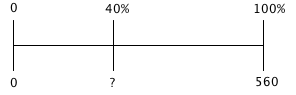Home > CC2MN > Chapter cc26 > Lesson cc26.2.3 > Problem6-82

6-82.

Forty percent of the students at Pinecrest Middle School have a school sweatshirt. There are $560$ students at the school. Draw a diagram to help you solve each problem below. Homework Help ✎

1. How many students have a school sweatshirt?$\frac{40}{100}\left(\frac{?}{?}\right)=\frac{\textit{x}}{560}$

$x=224$ students

2. If $280$ students have school t-shirts instead of sweatshirts, what percentage of the school has a t-shirt?

$280$ is what percent of $560$?

$\frac{\textit{x}}{100}\left(\frac{?}{?}\right)=\frac{280}{560}$

$x=50\%$

3. What percentage of the school does not have a t-shirt or a sweatshirt?

If $40\%$ of the students have a school sweatshirt and $50\%$ have a t-shirt instead of a sweatshirt, what is the remaining percentage of students that have neither?